## Disk Scheduling Algorithms-

Before you go through this article, make sure that you have gone through the previous article on Disk Scheduling.

We have discussed-

• Disk scheduling algorithms are used to schedule multiple requests for accessing the disk.
• The purpose of disk scheduling algorithms is to reduce the total seek time.

Various disk scheduling algorithms are-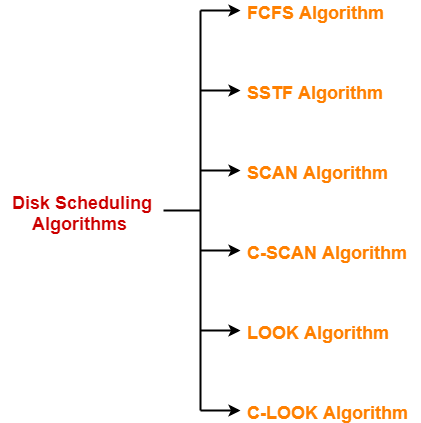In this article, we will discuss about C-LOOK Disk Scheduling Algorithm.

## C-LOOK Disk Scheduling Algorithm-

• Circular-LOOK Algorithm is an improved version of the LOOK Algorithm.
• Head starts from the first request at one end of the disk and moves towards the last request at the other end servicing all the requests in between.
• After reaching the last request at the other end, head reverses its direction.
• It then returns to the first request at the starting end without servicing any request in between.
• The same process repeats.

Also Read- SCAN Disk Scheduling Algorithm

• It does not causes the head to move till the ends of the disk when there are no requests to be serviced.
• It reduces the waiting time for the cylinders just visited by the head.
• It provides better performance as compared to LOOK Algorithm.
• It does not lead to starvation.
• It provides low variance in response time and waiting time.

• There is an overhead of finding the end requests.

Also Read- C-SCAN Disk Scheduling Algorithm

## Problem-01:

Consider a disk queue with requests for I/O to blocks on cylinders 98, 183, 41, 122, 14, 124, 65, 67. The C-LOOK scheduling algorithm is used. The head is initially at cylinder number 53 moving towards larger cylinder numbers on its servicing pass. The cylinders are numbered from 0 to 199. The total head movement (in number of cylinders) incurred while servicing these requests is _______.

## Solution-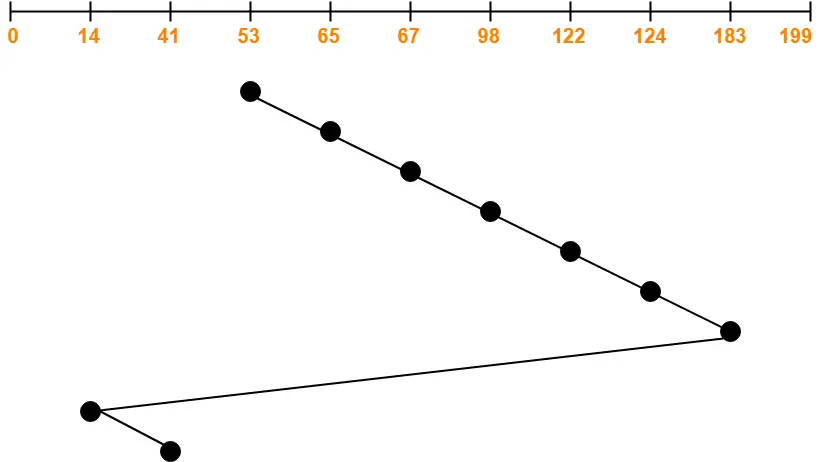Total head movements incurred while servicing these requests

= (65 – 53) + (67 – 65) + (98 – 67) + (122 – 98) + (124 – 122) + (183 – 124) + (183 – 14) + (41 – 14)

= 12 + 2 + 31 + 24 + 2 + 59 + 169 + 27

= 326

Alternatively,

Total head movements incurred while servicing these requests

= (183 – 53) + (183 – 14) + (41 – 14)

= 130 + 169 + 27

= 326

## Problem-02:

Consider a disk queue with requests for I/O to blocks on cylinders 47, 38, 121, 191, 87, 11, 92, 10. The C-LOOK scheduling algorithm is used. The head is initially at cylinder number 63 moving towards larger cylinder numbers on its servicing pass. The cylinders are numbered from 0 to 199. The total head movement (in number of cylinders) incurred while servicing these requests is _______.

## Solution-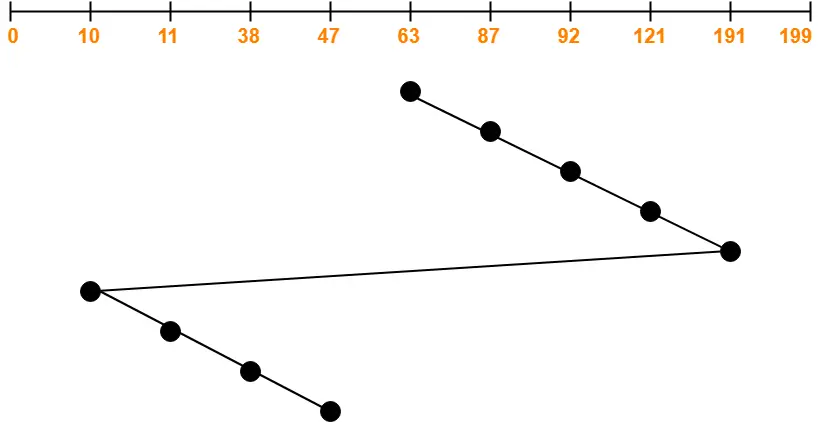Total head movements incurred while servicing these requests

= (87 – 63) + (92 – 87) + (121 – 92) + (191 – 121) + (191 – 10) + (11 – 10) + (38 – 11) + (47 – 38)

= 24 + 5 + 29 + 70 + 181 + 1 + 27 + 9

= 346

Alternatively,

Total head movements incurred while servicing these requests

= (191 – 63) + (191 – 10) + (47 – 10)

= 128 + 181 + 37

= 346

To gain better understanding about C-LOOK Disk Scheduling Algorithm,

Watch this Video Lecture

Get more notes and other study material of Operating System.

Watch video lectures by visiting our YouTube channel LearnVidFun.

## Disk Scheduling Algorithms-

Before you go through this article, make sure that you have gone through the previous article on Disk Scheduling.

We have discussed-

• Disk scheduling algorithms are used to schedule multiple requests for accessing the disk.
• The purpose of disk scheduling algorithms is to reduce the total seek time.

Various disk scheduling algorithms are-In this article, we will discuss about LOOK Disk Scheduling Algorithm.

## LOOK Disk Scheduling Algorithm-

• LOOK Algorithm is an improved version of the SCAN Algorithm.
• Head starts from the first request at one end of the disk and moves towards the last request at the other end servicing all the requests in between.
• After reaching the last request at the other end, head reverses its direction.
• It then returns to the first request at the starting end servicing all the requests in between.
• The same process repeats.

Also Read- C-SCAN Disk Scheduling Algorithm

## NOTE-

The main difference between SCAN Algorithm and LOOK Algorithm is-

• SCAN Algorithm scans all the cylinders of the disk starting from one end to the other end even if there are no requests at the ends.
• LOOK Algorithm scans all the cylinders of the disk starting from the first request at one end to the last request at the other end.

• It does not causes the head to move till the ends of the disk when there are no requests to be serviced.
• It provides better performance as compared to SCAN Algorithm.
• It does not lead to starvation.
• It provides low variance in response time and waiting time.

• There is an overhead of finding the end requests.
• It causes long waiting time for the cylinders just visited by the head.

## Problem-

Consider a disk queue with requests for I/O to blocks on cylinders 98, 183, 41, 122, 14, 124, 65, 67. The LOOK scheduling algorithm is used. The head is initially at cylinder number 53 moving towards larger cylinder numbers on its servicing pass. The cylinders are numbered from 0 to 199. The total head movement (in number of cylinders) incurred while servicing these requests is _______.

## Solution-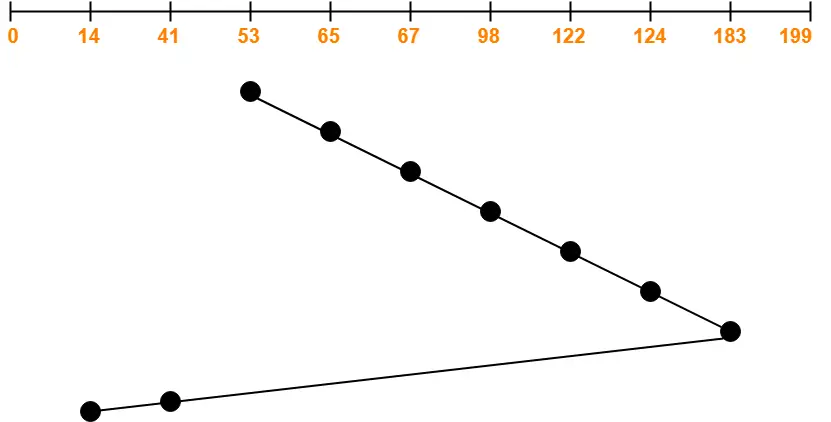Total head movements incurred while servicing these requests

= (65 – 53) + (67 – 65) + (98 – 67) + (122 – 98) + (124 – 122) + (183 – 124) + (183 – 41) + (41 – 14)

= 12 + 2 + 31 + 24 + 2 + 59 + 142 + 27

= 299

Alternatively,

Total head movements incurred while servicing these requests

= (183 – 53) + (183 – 14)

= 130 + 169

= 299

To gain better understanding about LOOK Disk Scheduling Algorithm,

Watch this Video Lecture

Next Article- C-LOOK Disk Scheduling Algorithm

Get more notes and other study material of Operating System.

Watch video lectures by visiting our YouTube channel LearnVidFun.

## Disk Scheduling Algorithms-

Before you go through this article, make sure that you have gone through the previous article on Disk Scheduling.

We have discussed-

• Disk scheduling algorithms are used to schedule multiple requests for accessing the disk.
• The purpose of disk scheduling algorithms is to reduce the total seek time.

Various disk scheduling algorithms are-In this article, we will discuss about C-SCAN Disk Scheduling Algorithm.

## C-SCAN Disk Scheduling Algorithm-

• Circular-SCAN Algorithm is an improved version of the SCAN Algorithm.
• Head starts from one end of the disk and move towards the other end servicing all the requests in between.
• After reaching the other end, head reverses its direction.
• It then returns to the starting end without servicing any request in between.
• The same process repeats.

Also Read- FCFS Disk Scheduling Algorithm

• The waiting time for the cylinders just visited by the head is reduced as compared to the SCAN Algorithm.
• It provides uniform waiting time.
• It provides better response time.

• It causes more seek movements as compared to SCAN Algorithm.
• It causes the head to move till the end of the disk even if there are no requests to be serviced.

Also Read- SSTF Disk Scheduling Algorithm

## Problem-

Consider a disk queue with requests for I/O to blocks on cylinders 98, 183, 41, 122, 14, 124, 65, 67. The C-SCAN scheduling algorithm is used. The head is initially at cylinder number 53 moving towards larger cylinder numbers on its servicing pass. The cylinders are numbered from 0 to 199. The total head movement (in number of cylinders) incurred while servicing these requests is _______.

## Solution-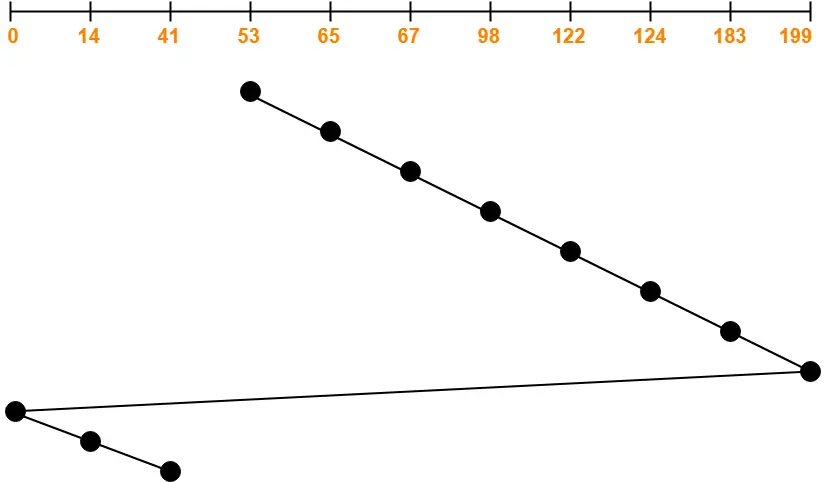Total head movements incurred while servicing these requests

= (65 – 53) + (67 – 65) + (98 – 67) + (122 – 98) + (124 – 122) + (183 – 124) + (199 – 183) + (199 – 0) + (14 – 0) + (41 – 14)

= 12 + 2 + 31 + 24 + 2 + 59 + 16 + 199 + 14 + 27

= 386

Alternatively,

Total head movements incurred while servicing these requests

= (199 – 53) + (199 – 0) + (41 – 0)

= 146 + 199 + 41

= 386

To gain better understanding about C-SCAN Disk Scheduling Algorithm,

Watch this Video Lecture

Next Article- LOOK Disk Scheduling Algorithm

Get more notes and other study material of Operating System.

Watch video lectures by visiting our YouTube channel LearnVidFun.

## Disk Scheduling Algorithms-

Before you go through this article, make sure that you have gone through the previous article on Disk Scheduling.

We have discussed-

• Disk scheduling algorithms are used to schedule multiple requests for accessing the disk.
• The purpose of disk scheduling algorithms is to reduce the total seek time.

Various disk scheduling algorithms are-In this article, we will discuss about SCAN Disk Scheduling Algorithm.

## SCAN Disk Scheduling Algorithm-

• As the name suggests, this algorithm scans all the cylinders of the disk back and forth.
• Head starts from one end of the disk and move towards the other end servicing all the requests in between.
• After reaching the other end, head reverses its direction and move towards the starting end servicing all the requests in between.
• The same process repeats.

## NOTE-

• SCAN Algorithm is also called as Elevator Algorithm.
• This is because its working resembles the working of an elevator.

Also Read- FCFS Disk Scheduling Algorithm

• It is simple, easy to understand and implement.
• It does not lead to starvation.
• It provides low variance in response time and waiting time.

• It causes long waiting time for the cylinders just visited by the head.
• It causes the head to move till the end of the disk even if there are no requests to be serviced.

Also Read- SSTF Disk Scheduling Algorithm

## Problem-

Consider a disk queue with requests for I/O to blocks on cylinders 98, 183, 41, 122, 14, 124, 65, 67. The SCAN scheduling algorithm is used. The head is initially at cylinder number 53 moving towards larger cylinder numbers on its servicing pass. The cylinders are numbered from 0 to 199. The total head movement (in number of cylinders) incurred while servicing these requests is _______.

## Solution-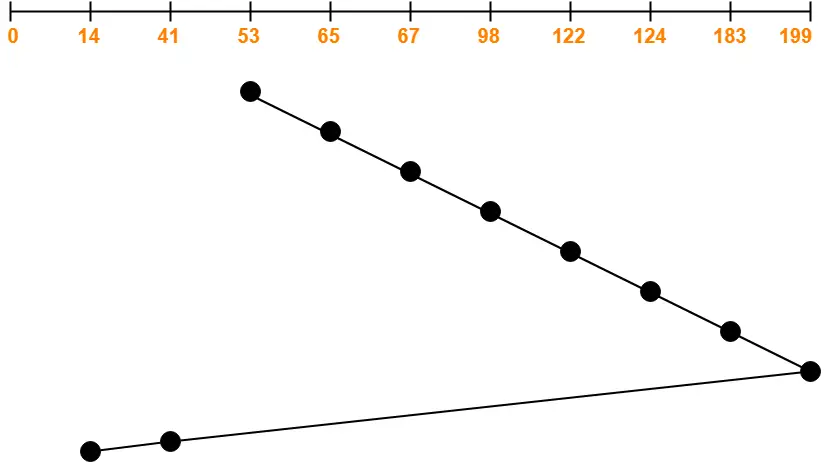Total head movements incurred while servicing these requests

= (65 – 53) + (67 – 65) + (98 – 67) + (122 – 98) + (124 – 122) + (183 – 124) + (199 – 183) + (199 – 41) + (41 – 14)

= 12 + 2 + 31 + 24 + 2 + 59 + 16 + 158 + 27

= 331

Alternatively,

Total head movements incurred while servicing these requests

= (199 – 53) + (199 – 14)

= 146 + 185

= 331

To gain better understanding about SCAN Disk Scheduling Algorithm,

Watch this Video Lecture

Next Article- C-SCAN Disk Scheduling Algorithm

Get more notes and other study material of Operating System.

Watch video lectures by visiting our YouTube channel LearnVidFun.

## Disk Scheduling Algorithms-

Before you go through this article, make sure that you have gone through the previous article on Disk Scheduling.

We have discussed-

• Disk scheduling algorithms are used to schedule multiple requests for accessing the disk.
• The purpose of disk scheduling algorithms is to reduce the total seek time.

Various disk scheduling algorithms are-In this article, we will discuss about SSTF Disk Scheduling Algorithm.

## SSTF Disk Scheduling Algorithm-

• SSTF stands for Shortest Seek Time First.
• This algorithm services that request next which requires least number of head movements from its current position regardless of the direction.
• It breaks the tie in the direction of head movement.

• It reduces the total seek time as compared to FCFS.
• It provides increased throughput.
• It provides less average response time and waiting time.

• There is an overhead of finding out the closest request.
• The requests which are far from the head might starve for the CPU.
• It provides high variance in response time and waiting time.
• Switching the direction of head frequently slows down the algorithm.

## Problem-01:

Consider a disk queue with requests for I/O to blocks on cylinders 98, 183, 41, 122, 14, 124, 65, 67. The SSTF scheduling algorithm is used. The head is initially at cylinder number 53 moving towards larger cylinder numbers on its servicing pass. The cylinders are numbered from 0 to 199. The total head movement (in number of cylinders) incurred while servicing these requests is _______.

## Solution-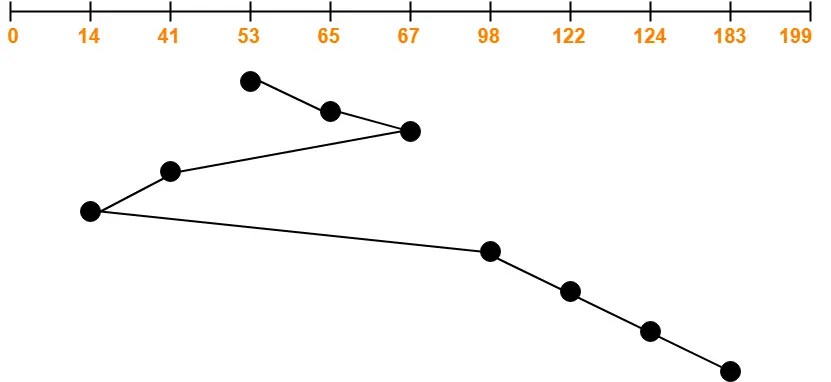Total head movements incurred while servicing these requests

= (65 – 53) + (67 – 65) + (67 – 41) + (41 – 14) + (98 – 14) + (122 – 98) + (124 – 122) + (183 – 124)

= 12 + 2 + 26 + 27 + 84 + 24 + 2 + 59

= 236

## Problem-02:

Consider a disk system with 100 cylinders. The requests to access the cylinders occur in following sequence-

4, 34, 10, 7, 19, 73, 2, 15, 6, 20

Assuming that the head is currently at cylinder 50, what is the time taken to satisfy all requests if it takes 1 ms to move from one cylinder to adjacent one and shortest seek time first policy is used?

1. 95 ms
2. 119 ms
3. 233 ms
4. 276 ms

## Solution-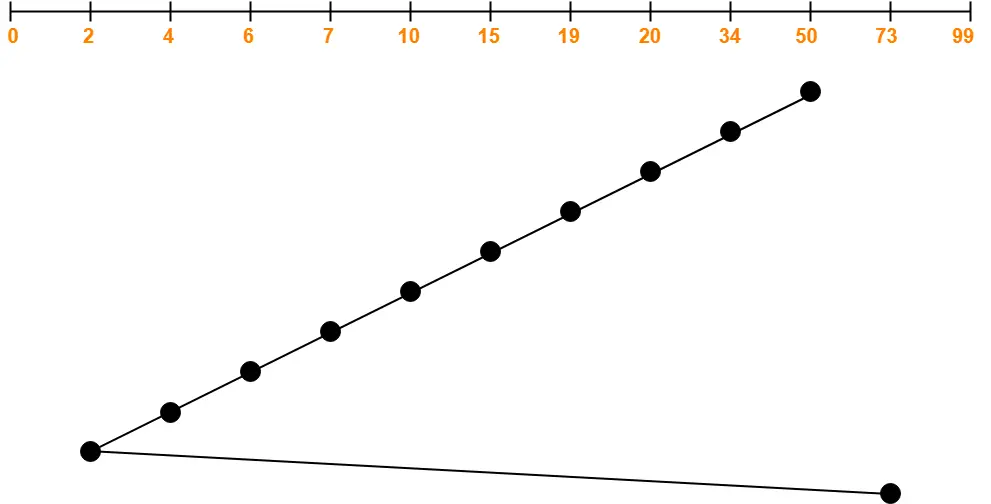Total head movements incurred while servicing these requests

= (50 – 34) + (34 – 20) + (20 – 19) + (19 – 15) + (15 – 10) + (10 – 7) + (7 – 6) + (6 – 4) + (4 – 2) + (73 – 2)

= 16 + 14 + 1 + 4 + 5 + 3 + 1 + 2 + 2 + 71

= 119

Time taken for one head movement = 1 msec. So,

Time taken for 119 head movements

= 119 x 1 msec

= 119 msec

Thus, Option (B) is correct.

To gain better understanding about SSTF Disk Scheduling Algorithm,

Watch this Video Lecture

Next Article- SCAN Disk Scheduling Algorithm

Get more notes and other study material of Operating System.

Watch video lectures by visiting our YouTube channel LearnVidFun.